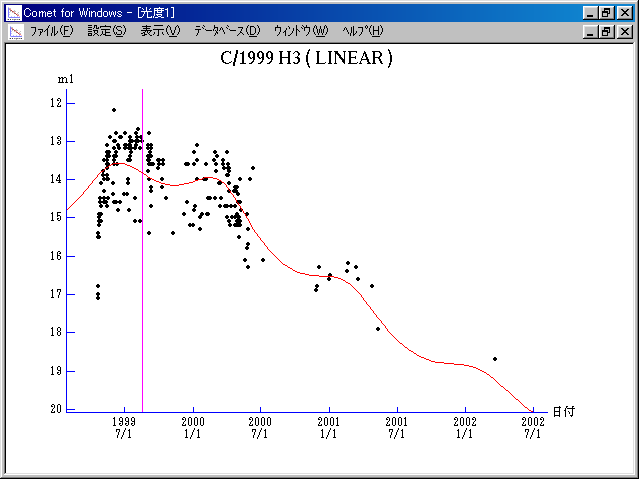# \$B%j%K%"WB@1(B

C/1999 H3 ( LINEAR )###\$B%W%m%U%#!<%k(B

 \$BH/8+F|(B 1999\$BG/(B 4\$B7n(B22\$BF|(B \$BH/8+8wEY(B 16.8\$BEy(B \$BH/8+ Lincoln Laboratory Near-Earth Asteroid Research project

###\$B###\$B50F;MWAG(B

```   The following improved orbital elements by Kenji Muraoka, are
from 786 observations 1999 Apr. 22 to 2001 Mar. 31, perturbations
by 9 Planets, Moon and 5 minor planets were taken into account.
The mean residual is +/- 0.71 arc seconds.

Epoch  =  1999 Aug. 10.0  TT       JDT = 2451400.5
T  =  1999 Aug. 18.23390       +/- 0.00029 (m.e.) TT
Peri. =  101.91125                +/- 0.00004
Node  =  332.72356                +/- 0.00004   (2000.0)
Incl. =  115.84027                +/- 0.00003
q  =    3.5008678              +/- 0.0000018 AU
e  =    1.0028222              +/- 0.0000027
1/a  =   -0.0008062              +/- 0.0000008 1/AU
orig. 1/a  =   +0.0000656
fut.  1/a  =   -0.0000092
```

###\$B@1?^(B###\$B8wEYJQ2=(B

```        m1 = 5.5 + 5 log\$B&\$(B + 10.0 log r
```##### \$B50F;MWAG\$OB<2,7r<#;a\$N7W;;\$K\$h\$k\$b\$N\$G\$9!#(B \$B@1?^\$O(B StellaNavigator Ver.2.0 for Windows (\$B%"%9%H%m%"!<%D(B \$BJTCx(B / \$B%"%9%-!<=PHG6I4)(B) \$B\$G:n@.\$7\$?\$b\$N\$G\$9!#(B \$B8wEY%0%i%U\$O(BComet for Windows\$B\$G:n@.\$7\$?\$b\$N\$G\$9!#(B